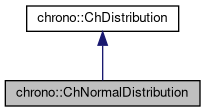chrono::ChNormalDistribution Class Reference

## Description

Class that generates the Gauss normal distribution (the 'bell' distribution) using the Box–Muller transform.

#include <ChDistribution.h>

Inheritance diagram for chrono::ChNormalDistribution:[legend]
Collaboration diagram for chrono::ChNormalDistribution:[legend]

## Public Member Functions

ChNormalDistribution (double mvariance, double mmean=0)
Create the normal distribution with assigned variance. More...

virtual double GetRandom () override
Compute a random value whose probability density is the normal distribution. More...

double GetMean () const

double GetVariance () constPublic Member Functions inherited from chrono::ChDistribution
virtual ~ChDistribution ()
Default destructor for distribution object.

## ◆ ChNormalDistribution()

 chrono::ChNormalDistribution::ChNormalDistribution ( double mvariance, double mmean = 0 )

Create the normal distribution with assigned variance.

The mean is 0 by default, but you can offset the curve if you want by providing a mean.

## ◆ GetRandom()

 double chrono::ChNormalDistribution::GetRandom ( )
overridevirtual

Compute a random value whose probability density is the normal distribution.

It uses the Box–Muller transform.

Implements chrono::ChDistribution.

The documentation for this class was generated from the following files:
• /builds/uwsbel/chrono/src/chrono/core/ChDistribution.h
• /builds/uwsbel/chrono/src/chrono/core/ChDistribution.cpp